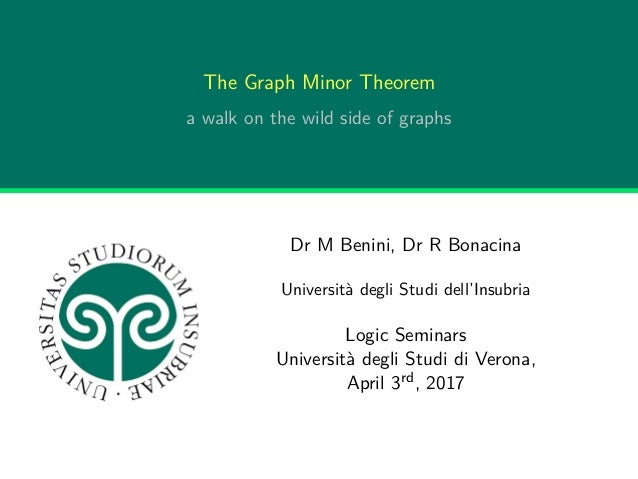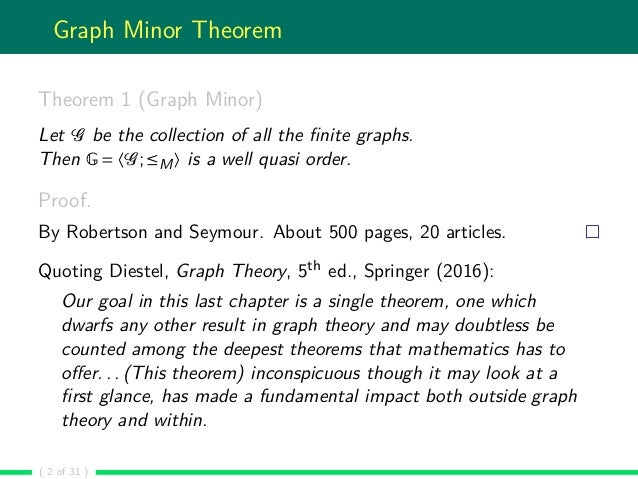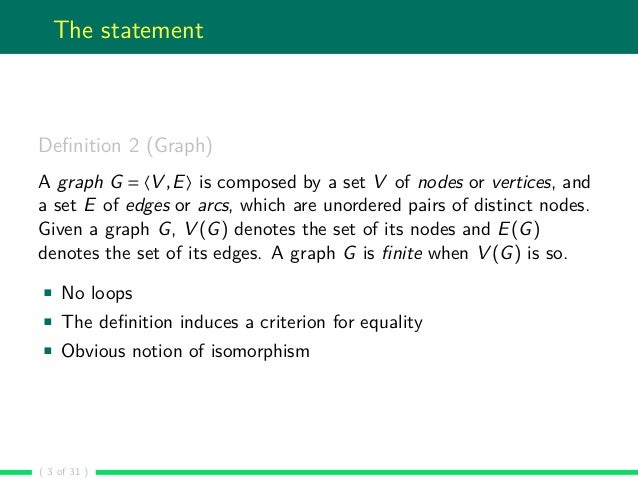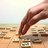Successfully reported this slideshow.Upcoming SlideShare
×

ofUpcoming SlideShare
Next

1 Like

Share

# The Graph Minor Theorem: a walk on the wild side of graphs

The Graph Minor Theorem says that the collection of finite graphs
ordered by the minor relation is a well quasi order. This apparently
innocent statement hides a monstrous proof: the original result by
Robertson and Seymour is about 500 pages and twenty articles, in which a
new and deep branch of Graph Theory has been developed.

The theorem is famous and full of consequences both on the theoretical side
of Mathematics and in applications, e.g., to Computer Science. But there
is no concise proof available, although many attempts have been made.
In this talk, arising from one such failed attempts, an analysis of the
Graph Minor Theorem is presented. Why is it so hard?

Assuming to use the by-now standard Nash-Williams's approach to prove it,we will
illustrate a number of methods which allow to solve or circumvent some
of the difficulties. Finally, we will show that the core of this line of
thought lies in a coherence question which is common to many parts of
Mathematics: elsewhere it has been solved, although we were unable to
adapt those solutions to the present framework. So, there is hope for a
short proof of the Graph Minor Theorem but it will not be elementary.

See all

See all

### The Graph Minor Theorem: a walk on the wild side of graphs

1. 1. The Graph Minor Theorem a walk on the wild side of graphs Dr M Benini, Dr R Bonacina Università degli Studi dell’Insubria Logic Seminars Università degli Studi di Verona, April 3rd, 2017
2. 2. Graph Minor Theorem Theorem 1 (Graph Minor) Let G be the collection of all the ﬁnite graphs. Then G = 〈G;≤M〉 is a well quasi order. Proof. By Robertson and Seymour. About 500 pages, 20 articles. Quoting Diestel, Graph Theory, 5th ed., Springer (2016): Our goal in this last chapter is a single theorem, one which dwarfs any other result in graph theory and may doubtless be counted among the deepest theorems that mathematics has to oﬀer. . . (This theorem) inconspicuous though it may look at a ﬁrst glance, has made a fundamental impact both outside graph theory and within. ( 2 of 31 )
3. 3. The statement Deﬁnition 2 (Graph) A graph G = 〈V ,E〉 is composed by a set V of nodes or vertices, and a set E of edges or arcs, which are unordered pairs of distinct nodes. Given a graph G, V (G) denotes the set of its nodes and E(G) denotes the set of its edges. A graph G is ﬁnite when V (G) is so. No loops The deﬁnition induces a criterion for equality Obvious notion of isomorphism ( 3 of 31 )
4. 4. The statement Deﬁnition 3 (Subgraph) G is a subgraph of H, G ≤S H, if and only if there is η: V (G) → V (H) injective such that, for every x,y ∈ E(G), η(x),η(y) ∈ E(H). Deﬁnition 4 (Induced subgraph) Let A ⊆ V (H). Then the induced subgraph G of H by A is identiﬁed by V (G) = A and E(G) = x,y ∈ E(H): x,y ∈ A . The notion of subgraph deﬁnes an embedding on graphs: G ≤S H says that there is a map η, the embedding, that allows to retrieve an image of G inside H. ( 4 of 31 )
5. 5. The statement Deﬁnition 5 (Path) Let G be a graph and let x,y ∈ V (G). A path p from x to y, p: x y, of length n ∈ N is a sequence vi ∈ V (G) 0≤i≤n such that (i) v0 = x, vn = y, (ii) for every 0 ≤ i < n, {vi ,vi+1} ∈ E(G), and (iii) for every 0 < i < j ≤ n, vi = vj. Deﬁnition 6 (Connected graph) A graph is connected when there is at least one path between every pair of nodes. ( 5 of 31 )
6. 6. The statement Deﬁnition 7 (Minor) G is a minor of H, G ≤M H, if and only if there is an equivalence relation ∼ on V (H) whose equivalence classes induce connected subgraphs in H, and G ≤S H/∼, with V (H/∼) = V (H)/∼ and E(H/∼) = [x]∼ ,[y]∼ : x ∼ y and x,y ∈ E(H) . For the sake of brevity, an equivalence inducing connected subgraphs as above, is called a c-equivalence. Fact 8 Let G be the collection of all the ﬁnite graphs. Then 〈G;≤S〉 and 〈G;≤M〉 are partial orders. ( 6 of 31 )
7. 7. The statement Deﬁnition 9 (Quasi order) A quasi order A = 〈A;≤〉 is a class A with a binary relation ≤ on A which is reﬂexive and transitive. If the relation is also anti-symmetric, A is a partial order. Given x,y ∈ A, x ≤ y means that x and y are not related by ≤; x is equivalent to y, x y, when x ≤ y and y ≤ x; x is incomparable with y, x y, when x ≤ y and y ≤ x. The notation x < y means x ≤ y and x y; x ≥ y is the same as y ≤ x; x > y stands for y < x. ( 7 of 31 )
8. 8. The statement Deﬁnition 10 (Descending chain) Let A = 〈A;≤〉 be a quasi order. Every sequence {xi ∈ A}i∈I, with I an ordinal, such that xi ≥ xj for every i < j is a descending chain. If a descending chain {xi }i∈I is such that xi > xj whenever i < j, then it is a proper descending chain. A (proper) descending is ﬁnite when the indexing ordinal I < ω. If every proper descending chain in A is ﬁnite, then the quasi order is said to be well founded. ( 8 of 31 )
9. 9. The statement Deﬁnition 11 (Antichain) Let A = 〈A;≤〉 be a quasi order. Every sequence {xi ∈ A}i∈I, with I an ordinal, such that xi xj for every i = j is an antichain. An antichain is ﬁnite when the indexing ordinal I < ω. If every antichain in A is ﬁnite, then the quasi order is said to satisfy the ﬁnite antichain property or, simply, to have ﬁnite antichains. Deﬁnition 12 (Well quasi order) A well quasi order is a well founded quasi order having the ﬁnite antichain property. ( 9 of 31 )
10. 10. Nash-Williams’s toolbox Deﬁnition 13 (Bad sequence) Let A = 〈A;≤〉 be a quasi order. An inﬁnite sequence {xi }i∈ω in A is bad if and only if xi ≤ xj whenever i < j. A bad sequence {xi }i∈ω is minimal in A when there is no bad sequence yi i∈ω such that, for some n ∈ ω, xi = yi when i < n and yn < xn. In fact, in the following, a generalised notion of ‘being minimal’ is used: a bad sequence {xi }i∈ω is minimal with respect to µ and r in A when for every bad sequence yi i∈ω such that, for some n ∈ ω, xi r yi when i < n , it holds that µ(yn) <W µ(xn). Here, µ: A → W is a function from A to some well founded quasi order 〈W ;≤W 〉 and r is a reﬂexive binary relation on A. ( 10 of 31 )
11. 11. Nash-Williams’s toolbox Theorem 14 Let A = 〈A;≤〉 be a quasi order. Then, the following are equivalent: 1. A is a well quasi order; 2. in every inﬁnite sequence {xi }i∈ω in A there exists an increasing pair xi ≤ xj for some i < j; 3. every sequence {xi ∈ A}i∈ω contains an increasing subsequence xnj j∈ω such that xni ≤ xnj for every i < j. 4. A does not contain any bad sequence. There is also a ﬁnite basis characterisation which is of interest because it has been used to prove the Graph Minor Theorem. But we will not illustrate it today. ( 11 of 31 )
12. 12. Nash-Williams’s toolbox Lemma 15 (Dickson) Assume A and B to be non empty sets. Then A = 〈A;≤A〉 and B = 〈B;≤B〉 are well quasi orders if and only if A×B = 〈A×B;≤×〉 is a well quasi order, with the ordering on the Cartesian product deﬁned by (x1,y1) ≤× (x2,y2) if and only if x1 ≤A x2 and y1 ≤B y2. ( 12 of 31 )
13. 13. Nash-Williams’s toolbox Let A = 〈A;≤A〉 be a well quasi order, and deﬁne A∗ = {xi }i<n : n ∈ ω and, for all i < n,xi ∈ A as the collection of all the ﬁnite sequences over A. Then A∗ = 〈A∗ ;≤∗〉 is deﬁned as {xi }i<n ≤∗ yi i<m if and only if there is η: n → m injective and monotone between the ﬁnite ordinals n and m such that xi ≤A yη(i) for all i < n. Lemma 16 (Higman) A∗ = 〈A∗ ;≤∗〉 is a well quasi order. Dropping the requirement that η above has to be monotone, leads to a similar result. ( 13 of 31 )
14. 14. Nash-Williams’s toolbox Theorem 17 (Kruskal) Let T be the collection of all the ﬁnite trees. Then T = 〈T ;≤M〉 is a well quasi order. We remind that a tree is a connected and acyclic graph. Kruskal’s Theorem has much more to reveal than its statement says: for example, it is unprovable in Peano arithmetic. ( 14 of 31 )
15. 15. Nash-Williams’s toolbox Lemma 18 Let A = 〈A;≤A〉 be a quasi order which is not a well quasi order, and let 〈W ;≤〉 be a well founded quasi order. Also, let f : A → W be a function and r ⊆ A×A a reﬂexive relation. Then, there is a bad sequence {xi }i∈ω on A that is minimal with respect to f and r: for every n ∈ ω and for every bad sequence yi i∈ω on A such that xi r yi whenever i < n, f (yn) < f (xn). ( 15 of 31 )
16. 16. Nash-Williams’s toolbox Let B = 〈B;≤B〉 be a quasi order. Let 〈W ;≤〉 be a total well founded quasi order, and let µ: B → W be a function. Also, let r be a reﬂexive relation on B, which is used to possibly identify distinct elements in B. Suppose B is not a well quasi order, then there is {Bi }i∈ω bad in B and minimal with respect to µ and r by Lemma 18. Let p ∈ ω and let ∆: Bi : i ≥ p → ℘ﬁn(B), the collection of all the ﬁnite subsets of B, be such that (∆1) for every i ∈ ω and for every x ∈ ∆(Bi ), x ≤B Bi ; (∆2) for every i ∈ ω and for every x ∈ ∆(Bi ), µ(x) < µ(Bi ). Proposition 19 Let D = 〈 i>p ∆(Bi );≤B〉. Then D is a well quasi order. ( 16 of 31 )
17. 17. Nash-Williams’s toolbox Summarising, we want to prove that B = 〈B;≤B〉 is a well quasi order, and we know it is a quasi order. Suppose B is not a well quasi order. Then there is a minimal bad sequence {Bi }i∈ω with respect to some reasonable measure µ and =. Deﬁne a decomposition ∆ of the elements in the bad sequence. Then, the collection of the components forms a well quasi order. Form a sequence C from the components: by using the previous results (Dickson, Higman, Kruskal, and variants) it is usually easy to deduce that C lies in a well quasi order. Then, C contains an increasing pair. So, each component of Bn is less than a component in Bm. Recombine the pieces, and it follows (!) that Bn ≤ Bm, contradicting the initial assumption. Q.E.D. ( 17 of 31 )
18. 18. A non-proof of GMT We want to prove that G = 〈G;≤M〉 is a well quasi order, and we know it is a quasi order. Suppose G is not a well quasi order. Then there is a minimal bad sequence {Bi }i∈ω with respect to E(_) and =. Fix a sequence ei ∈ E(Bi ) i>p and deﬁne ∆(Bi ) = G : V (G ) = V (G) and E(G ) = E(G){ei } . It is easy to check that there is ﬁnite number of components; each component is a minor of Bi ; each component has less edges than Bi ; for some p ∈ ω, each Bi , i > p, contains an arc. Then, the collection of the components D forms a well quasi order. Construct the sequence C = ∆(Bi ) i>p: it lies in D. Then, C contains an increasing pair Bn ≤M Bm Add back the arcs en and em. Thus Bn ≤M BM. Contradiction. ( 18 of 31 )
19. 19. A non-proof of GMT The red part is wrong, already on forests: M but = When adding back edges, they are linked to the ‘wrong’ nodes, preventing to lift the embedding on the parts to the whole. ( 19 of 31 )
20. 20. Embeddings versus minors G ≤M H means that there is an embedding of G into H: a map f from the nodes of G to a quotient on the nodes of H by means of a c-equivalence such that f preserves arcs. The problem in the previous non-proof is that the cancelled edges eG and eH in the decomposition process lie between two nodes which may be mapped by f in such a way that f (eG) = eH. But, if one is able to constrain the way nodes are mapped. . . ( 20 of 31 )
21. 21. Back to Kruskal In the case of Kruskal’s Theorem 17, the problem is easy to solve: deleting an arc yields two disjoint subtrees. Considering them as two distinct components does not change the pattern of the proof. But marking the root and using a minor relation ≤R which preserves roots, is the key to a concise and elegant proof. In fact, it suﬃces to show that the restricted minor relation ≤R is contained in the usual ≤M to derive the theorem: in fact, if there is a bad sequence {Bi }i∈ω with respect to ≤M, then it is bad with respect to ≤R. So, if 〈T ;≤R〉 is a well quasi order, then it cannot contain a bad sequence, and so neither can 〈T ;≤M〉. ( 21 of 31 )
22. 22. Coherence The Kruskal Theorem is very nice subcase of the Graph Minor Theorem, which points to the core of the problem. It is not the independence of the components, like in Kruskal’s case, that marks the diﬀerence. Rather, it is the fact that we can identify a sub-relation of ≤M which preserves roots. And this is enough to prove Kruskal’s Theorem. It is tempting to extend the idea behind Kruskal’s Theorem to general graphs. And it is easy: mark the endpoints of the arcs we are going to delete. Formally, this amounts to prove the Graph Minor Theorem on labelled graphs, with a few additional hypotheses. And it suﬃces to prove this extended theorem for labellings over the well quasi order 2. The proof develops smoothly as before. . . and, frustratingly, it fails in the same point. ( 22 of 31 )
23. 23. Coherence We have tried many variations on the theme, and we learnt how to move the problem in diﬀerent parts of the proof. But, still, we have not found how to solve it. The nature of the problem is subtle: it is about the coherence of embeddings. It is easier to explain it by means of an example: M = ( 23 of 31 )
24. 24. Coherence The blue arrows denote the embedding as obtained from the decomposition step. The red arrows denote the embedding of the cancelled arc on the left to the cancelled arc on the right. The green edge is the embedding of the red arc on the left as a result of the blue embedding. The blue and the red embedding are not coherent: they to not preserve the endpoints of arcs. = ( 24 of 31 )
25. 25. Coherence Coherence can be described in an abstract way: G G G ... H H H ... f f F Coherence amounts to require that there is an embedding F such that the above diagram commutes. The f arrows are suitable embeddings of the components G of G into the components H of H. When this happens, we say that the f ’s are coherent, since they can be derived by factoring F through the inclusions of components. ( 25 of 31 )
26. 26. Coherence G G G ... H H H ... f f F In a way, this diagram suggests to construct a colimit in a suitable category, the one of graphs and coherent embeddings. It is exactly the way to synthesise the proof of Kruskal’s Theorem: look for a relation which forces G to be the minimal object containing all the components, and such that the considered embeddings can be combined together, so to construct the F above, which acts as the co-universal arrow. ( 26 of 31 )
27. 27. Hopes for the future The illustrated approach almost yields a proof of the Graph Minor Theorem, except for solving the coherence problem. It is worth remarking that this problem is not about graph, but about embeddings: we need a way to identify which embeddings can be combined so to form a co-universal arrow as in the preceding diagram, and we need to show that the minor relation arising from them is contained in ≤M. Then, as in the Kruskal’s case, the result follows. But this fact gives hopes to ﬁnd a concise proof of the Graph Minor Theorem: the coherence problem arises in many branches of Mathematics, completely unrelated to Graph Theory. And many solutions have been found. As soon as one identiﬁes that a theorem in the X theory provides a solution to an instance of the abstract coherence problem, there are chances it could be reused to write Q.E.D. after the proof we have illustrated before. ( 27 of 31 )
28. 28. A minor surprise Let G be a ﬁnite graph: deﬁne g(G) = (x,y) : x,y ∈ E(G) ∪ (x,x) : x ∈ V (G) . Then g(G) is a ﬁnite reﬂexive and symmetric relation over a ﬁnite set. Conversely, if γ is a ﬁnite reﬂexive and symmetric relation over a ﬁnite set, deﬁne g−1(γ) as the graph G such that V (G) = x : x γ x and E(G) = x,y : x γ y and x = y . Clearly g and g−1 are each other inverses. Thus, a graph can be seen as a ﬁnite, reﬂexive and symmetric relation. ( 28 of 31 )
29. 29. A minor surprise If G ≤S H then there is η: V (G) → V (H) injective such that, for every x,y ∈ E(G), η(x),η(y) ∈ E(H). there is η injective such that, whenever (x,y) ∈ g(G), (η(x),η(y)) ∈ g(H), that is, there is a pointwise monomorphism g(G) → g(H). Suppose ∼ to be a c-equivalence on H, then ∼ is an equivalence relation and, for every x ∈ V (H), the subgraph of H induced by [x]∼ is connected. ∼ is an equivalence relation and, for every x in the domain dom(g(H)) of the relation g(H), every pair of elements in [x]∼ lie in g(H)∗ , the transitive closure of g(H). ∼ is an equivalence on dom(g(H)) such that ∼ ⊆ g(H)∗ . ( 29 of 31 )
30. 30. A minor surprise Hence, G ≤M H if and only if there is an equivalence relation ∼ on dom(g(H)) such that ∼ ⊆ g(H)∗ , and there is η: dom(g(G)) → dom(g(H))/∼ injective such that (η(x),η(y)) : (x,y) ∈ g(G) = η(g(G)) ⊆ g(H)/∼ = ([x]∼,[y]∼) : (x,y) ∈ g(H) . Let γ and δ be ﬁnite, reﬂexive and symmetric relations. Deﬁne γ ≤R δ if and only if there is an equivalence ∼ on dom(δ) such that ∼ ⊆ δ∗ , and there is η: dom(γ) → dom(δ)/∼ injective such that η(γ) ⊆ δ/∼. Theorem 20 (Graph Minor) Let R be the collection of ﬁnite, reﬂexive and symmetric relations. Then R = 〈R;≤R〉 is a well quasi order. So, surprise, the Graph Minor Theorem is not about graphs! ( 30 of 31 )
31. 31. The end Questions? ( 31 of 31 )
•#### mogproject

Apr. 21, 2019

Total views

266

On Slideshare

0

From embeds

0

Number of embeds

1

2

Shares

0